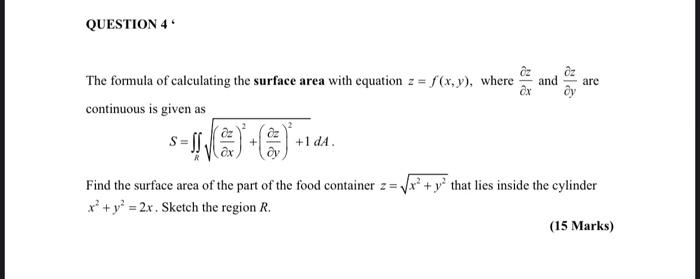### Create an Account

Home / Questions / QUESTION 4 and are The formula of calculating the surface area with equation z = f(x,y), w...

# QUESTION 4 and are The formula of calculating the surface area with equation z = f(x,y), where continuous is given as +1 DA Find the surface area of the part of the food container z= Vx+y that lies in

QUESTION 4 and are The formula of calculating the surface area with equation z = f(x,y), where continuous is given as +1 DA Find the surface area of the part of the food container z= Vx+y that lies inside the cylinder x2 + y2 = 2x. Sketch the region R. (15 Marks)May 08 2021 View more View LessSubscribe To Get Solution